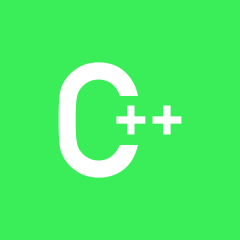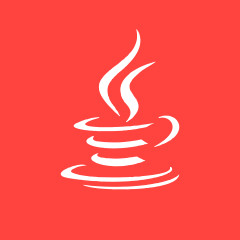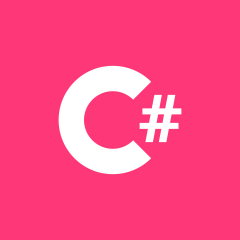C++C++ CC++ CC++ CC++ 源码C++ PHPC++ C
c++ 用GetCommandLine（） 获取命令行参数出现中文乱码，怎么办？C++ C
java里Runtime.getRuntime("cmd /c start......")问题，如下JAVA C++C C++操作系统 设计模式 C++C++ PHPC++ CC++ CC++C C++JAVA C C++C++ CC++ CC++ PHPC++ C PHPC++ PHPC# C++C++ CC++ CC C++ C#C C# C++C C++ JavaScriptC++ Html/CSS JavaScript
C编写程序实数取整C++ C#C++ C#